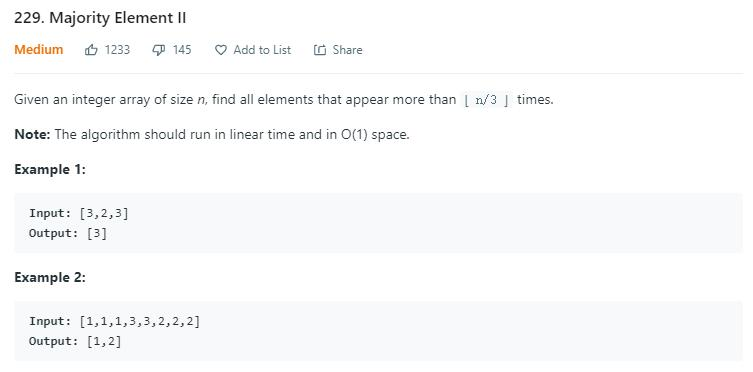# 题目描述（中等难度）# 解法一

map 的话 key 存数字，value 存数字出现的个数。如果数字出现的次数等于了 n/3 + 1 就把它加到结果中。

public List<Integer> majorityElement(int[] nums) {
int n = nums.length;
HashMap<Integer, Integer> map = new HashMap<>();
List<Integer> res = new ArrayList<>();
for (int i = 0; i < n; i++) {
int count = map.getOrDefault(nums[i], 0);
//之前的数量已经是 n/3, 当前数量就是 n/3 + 1 了
if (count == n / 3) {
}
//可以提前结束
if (count == 2 * n / 3 || res.size() == 2) {
return res;
}
map.put(nums[i], count + 1);

}
return res;
}


# 解法二

169 题 我们做过找出数组的中超过 n/2 数量的数字，其中介绍了摩尔投票法，这里的话可以改写一下，参考 这里

group 记录当前队伍的人数，count 记录当前队伍剩余的人数。如果当前队伍剩余人数为 0，记录下次遇到的人的所在队伍号。

group1group2 首先初始化为不可能和当前数字相等的两个数，将这两个队伍看成同盟，它俩不互相伤害。

public List<Integer> majorityElement(int[] nums) {
int n = nums.length;
long group1 = (long)Integer.MAX_VALUE + 1;
int count1 = 0;
long group2 = (long)Integer.MAX_VALUE + 1;
int count2 = 0;
for (int i = 0; i < n; i++) {
if (nums[i] == group1) {
count1++;
} else if (nums[i] == group2) {
count2++;
} else if (count1 == 0) {
group1 = nums[i];
count1 = 1;
} else if (count2 == 0) {
group2 = nums[i];
count2 = 1;
} else {
count1--;
count2--;
}
}

//计算两个队伍的数量,因为可能只存在一个数字的数量超过了 n/3
count1 = 0;
count2 = 0;
for (int i = 0; i < n; i++) {
if (nums[i] == group1) {
count1++;
}
if (nums[i] == group2) {
count2++;
}
}
//只保存数量大于 n/3 的队伍
List<Integer> res = new ArrayList<>();
if (count1 > n / 3) {
}

if (count2 > n / 3) {
}
return res;
}


2020.5.27 更新，@Frankie 提醒，其实不用上边分析的那么麻烦，只需要给 group1group2 随便赋两个不相等的值即可。

public List<Integer> majorityElement(int[] nums) {
int n = nums.length;
int group1 = 0;
int count1 = 0;
int group2 = 1;
int count2 = 0;
for (int i = 0; i < n; i++) {
if (nums[i] == group1) {
count1++;
} else if (nums[i] == group2) {
count2++;
} else if (count1 == 0) {
group1 = nums[i];
count1 = 1;
} else if (count2 == 0) {
group2 = nums[i];
count2 = 1;
} else {
count1--;
count2--;
}
}

//计算两个队伍的数量,因为可能只存在一个数字的数量超过了 n/3
count1 = 0;
count2 = 0;
for (int i = 0; i < n; i++) {
if (nums[i] == group1) {
count1++;
}
if (nums[i] == group2) {
count2++;
}
}
//只保存数量大于 n/3 的队伍
List<Integer> res = new ArrayList<>();
if (count1 > n / 3) {
}

if (count2 > n / 3) {